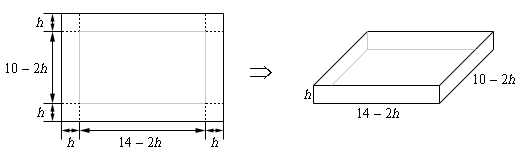# Generating Box ProblemsIt's likely if you're reading this webpage that you are either taking calculus now, or have taken calculus in the past. Depending on how good your memory is you may remember doing this sort of problem many times.

It turns out that this problem has been in use for over a hundred years! Google Books even has a book from 1855 by Issac Todhunter with a version of this problem! It's number 29, you may have to scroll up to see it.

Suppose now that you're a teacher and you like giving this sort of problem to students. Suppose you even like giving problems whose solutions are rational numbers. I certainly do! Well here's a way to generate lots and lots of such problems. In fact, if you hit evaluate and go through all possible denominators in the code below you get every possible rational box problem! The parameter t determines where between 0 and 1 your point is on the y axis, while x and y are the dimensions of the piece of cardboard you start with!